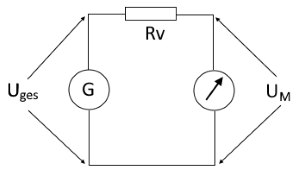# Series resistance for a voltmeter

Calculating the series resistance to extend the measuring range of an analog voltmeter

## Voltmeter measuring range extension

This function calculates a series resistor to extend the measuring range of an analog voltmeter. In principle, the calculation can also be used for any other component, whose supply voltage is to be reduced.The voltmeter voltage and the total voltage must be known for the calculation. In addition, the current or resistance of the voltmeter must be specified. The input of the internal resistance of the voltmeter is preset; it can be switched to the current with the pull-down menu.

Voltmeter series resistor calculator

 Input Total voltage mV V Voltmeter voltage mV V Voltmeter resistance Voltmeter current mΩ Ω kΩ MΩ mA A Decimal places 0 1 2 3 4 6 8 10 Result Series resistor Power of the Rv

## Formulas for series resistance

#### Formula for calculating the series resistance using the voltmeter current

$$\displaystyle R_v=\frac{U_{ges}-U_m}{I_m}$$

#### Calculation of the series resistance via the voltmeter resistance

$$\displaystyle R_v=\left(\frac{U_{ges}}{U_m}-1\right)*R_m$$

#### Legend

 $$\displaystyle U_{ges}$$ Total voltage / input voltage $$\displaystyle U_{m}$$ Voltage on voltmeter $$\displaystyle R_{m}$$ Resistance of the voltmeter $$\displaystyle I_{m}$$ Current in voltmeter $$\displaystyle R_{v}$$ Resistance of the series resistor $$\displaystyle P_{v}$$ Power of the series resistor

 Ist diese Seite hilfreich?             Vielen Dank für Ihr Feedback! Wie können wir die Seite verbessern?